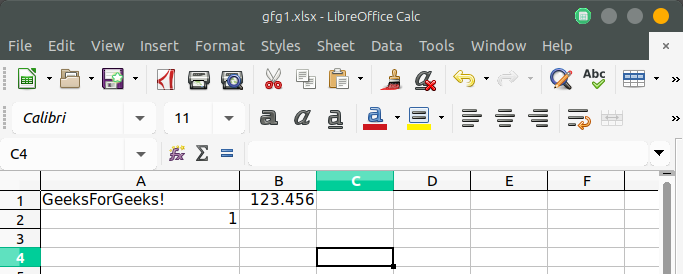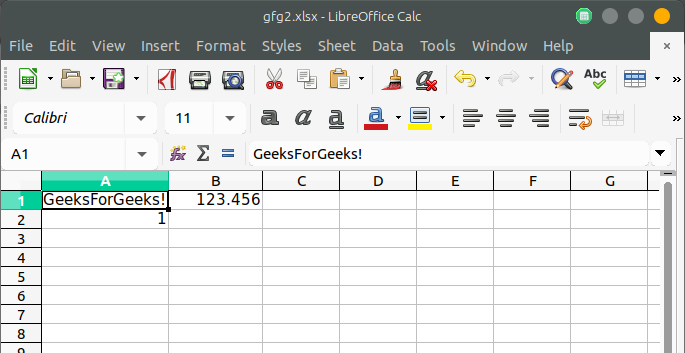Related Articles
PHP | Spreadsheet | Setting a cell value by coordinate
• Difficulty Level : Basic
• Last Updated : 19 Nov, 2018

The setCellValue() function is an inbuilt function in PHPSpreasheet which is used to set the value of a cell in a spreadsheet.

Syntax:

`setCellValue( \$coordinate, \$value )`

Parameters: This function accepts two parameters as mentioned above and described below:

• \$coordinate: This parameter is used to store the value of the coordinate of the excel sheet.
• \$value: This parameter is used to store the value of data which is to be set in excel sheet.

Return Value: This function returns Object of class PhpOffice\PhpSpreadsheet\Worksheet\Worksheet.

Example 1:

 `getActiveSheet();  ` ` `  `// Sets cell A1 with String Value  ` `\$sheet``->setCellValue(``'A1'``, ``'GeeksForGeeks!'``);  ` ` `  `// Sets cell A2 with Boolean Value  ` `\$sheet``->setCellValue(``'A2'``, TRUE);  ` ` `  `// Sets cell B1 with Numeric Value  ` `\$sheet``->setCellValue(``'B1'``, 123.456);  ` ` `  `// Write an .xlsx file  ` `\$writer` `= ``new` `Xlsx(``\$spreadsheet``);  ` ` `  `// Save .xlsx file to the current directory ` `\$writer``->save(``'gfg1.xlsx'``);  ` `?> `

Output:Alternatively, It can be achieved by retrieving the cell object firstly and then set the value. For this purpose, Cell object can be retrieved with the help of getCell() function and then the value can be set with the help of setValue() function.
Syntax:

`\$spreadsheet->getActiveSheet()->getCell(\$coordinate)->setValue(\$value);`

Example 2:

 `getActiveSheet();  ` ` `  `// Sets cell A1 with String Value  ` `\$sheet``->getCell(``'A1'``)->setValue(``'GeeksForGeeks!'``); ` ` `  `// Sets cell A2 with Boolean Value  ` `\$sheet``->getCell(``'A2'``)->setValue(TRUE); ` ` `  `// Sets cell B1 with Numeric Value  ` `\$sheet``->getCell(``'B1'``)->setValue(123.456); ` ` `  `// Write an .xlsx file  ` `\$writer` `= ``new` `Xlsx(``\$spreadsheet``); ` ` `  `// Save .xlsx file to the current directory ` `\$writer``->save(``'gfg2.xlsx'``);  ` `?> `

Output:My Personal Notes arrow_drop_up
Recommended Articles
Page :